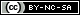# Weibull++ Examples

(Difference between revisions)
 Revision as of 21:11, 18 April 2014 (view source)← Older edit Revision as of 15:34, 13 June 2014 (view source)Newer edit → Line 1: Line 1: - {{Allexamplesindex}}[[Image:Weibull_Examples_Banner.png|left|400px|link=Weibull++_Examples|alt=Weibull++ Examples|Caption]] [[Image:Weibullbox.png|100px|right|link=Life_Data_Analysis_Reference_Book]]__NOTOC__ + {{Allexamplesindex}}[[Image:Weibull_Examples_Banner.png|left|400px|link=Weibull++_Examples|alt=Weibull++ Examples|Caption]] [[Image:Weibullbox.png|100px|right|link=Life_Data_Analysis_Reference_Book]]__NOTOC__[[Category:Index Pages]] Line 16: Line 16: ==Parametric Life Data Analysis== ==Parametric Life Data Analysis== - *Simple Probability Plotting Examples: [[Probability Plotting Example|Simple Example]], [[3-Parameter_Weibull_Example|3-P Weibull Example]], [[1P_Exponential_Example|1-P Exponential]], [[Normal Distribution Probability Plotting Example|Normal]], [[Example:_Lognormal_Distribution_Probability_Plot|Lognormal]] + *Simple Probability Plotting Examples: [[Probability Plotting Example|Simple Example]], [[3-Parameter_Weibull_Example|3P-Weibull Example]], [[1P_Exponential_Example|1P-Exponential]], [[Normal Distribution Probability Plotting Example|Normal]], [[Example:_Lognormal_Distribution_Probability_Plot|Lognormal]] *[[Standard_Folio_Plots|Standard Folio Plots]] *[[Standard_Folio_Plots|Standard Folio Plots]] *Examples by Distribution: *Examples by Distribution:

## Quick Start Guide

The Weibull++ & ALTA Quick Start Guide has been designed to help you explore many of the software's key features by working through step-by-step instructions for some practical application examples. The guide is available as a free *.PDF download and you can also watch videos posted on ReliaSoft.tv.

## Non-Parametric Life Data Analysis

• Crack Propagation Example (Point Estimation). View it in HTML or Video

## UtilitiesContent on this site is available/licensed under the "Creative Commons Attribution-NonCommercial-ShareAlike 4.0 International License." See Copyright Information for details.